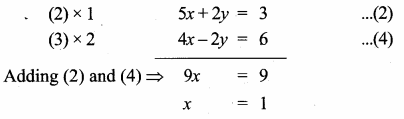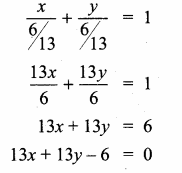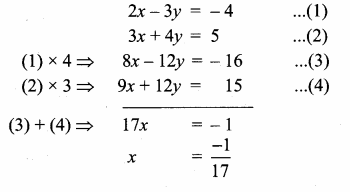1. Home
2. /
3. Blog
4. /
6. /
7. 10th Standard
8. /
9. Maths
10. /
11. Chapter 5
12. /
13. Chapter 5 -Coordinate Geometry,...

# Chapter 5 -Coordinate Geometry, Unit Exercise 5,

10 Dec 2020 3:58 pm

Tamilnadu, Samacheer, Kalvi, 10th, sslc, Maths, Solutions, Chapter 5, Coordinate Geometry, Unit Exercise 5,

Question 1.
PQRS is a rectangle formed by joining the points P(- 1, – 1), Q(- 1, 4) , R(5, 4) and S (5, – 1). A, B, C and D are the mid-points of PQ, QR, RS and SP respectively. Is the quadrilateral ABCD a square, a rectangle or a rhombus? Justify your answer.

Mid point of a line = ([Math Processing Error])
Mid point of PQ (A) = ([Math Processing Error],[Math Processing Error])
= ([Math Processing Error],[Math Processing Error]) = (-1,[Math Processing Error])
Mid point of QR (B) = ([Math Processing Error],[Math Processing Error]) = ([Math Processing Error],[Math Processing Error]) = (2,4)
Mid point of RS (C) = ([Math Processing Error],[Math Processing Error]) = ([Math Processing Error],[Math Processing Error]) = (5,[Math Processing Error])
Mid point of PS (D) = ([Math Processing Error],[Math Processing Error]) = ([Math Processing Error],[Math Processing Error]) = (2,-1)

img 355
AB = BC = CD = AD = [Math Processing Error]
Since all the four sides are equal,
∴ ABCD is a rhombus.

Question 2.
The area of a triangle is 5 sq. units. Two of its vertices are (2,1) and (3, -2). The third vertex is (x, y) where y = x + 3 . Find the coordinates of the third vertex.
Let the vertices A(2,1), B(3, – 2) and C(x, y)
Area of a triangle = 5 sq. unit

[Math Processing Error] [x1y2 + x2y3 + x3y1– (x2y1 + x3y2 + x1y3)] = 5
[Math Processing Error] [-4 + 3y + x – (3 – 2x + 2y)] = 5
-4 + 3y + x – 3 + 2x – 2y = 10
3x + y – 7 = 10
3x + y = 17 ……(1)
Given y = x + 3
Substitute the value ofy = x + 3 in (1)
3x + x + 3 = 17
4x = 17 – 3
4x = 14
x = [Math Processing Error] = [Math Processing Error]
Substitute the value of x in y = x + 3
y = [Math Processing Error] + 3 ⇒ y = [Math Processing Error] = [Math Processing Error]
∴ The coordinates of the third vertex is ([Math Processing Error],[Math Processing Error])

www.asterclasses.com

Question 3.
Find the area of a triangle formed by the lines 3x + y – 2 = 0, 5x + 2y – 3 = 0 and 2x – y – 3 = 0
3x + y = 2 ……..(1)
5x + 2y = 3 ………(2)
2x – y = 3 ……….(3)
Solve (1) and (2) to get the vertices Bp

Substitute the value of x = 1 in (1)
3(1) + y = 2
y = 2 – 3 = – 1
The point B is (1, – 1)
Solve (2) and (3) to get the vertices CSubstitute the value of x = 1 in (3)
2(1) – y = 3 ⇒ -y = 3 – 2
– y = 1 ⇒ y = – 1
The point C is (1, – 1)
Solve (1) and (3) to get the vertices ASubstitute the value of x = 1 in (1)
3(1) + y = 2
y = 2 – 3 = -1
The point A is (1, – 1)
The points A (1, – 1), B (1, -1), C(1, -1)
Area of ∆ABC = [Math Processing Error]

[x1y2 + x2y3 + x3y1 – (x2y1 + x3y2 + x1y3)]

Area of the triangle = 0 sq. units.
Note: All the three vertices are equal, all the point lies in a same points.

www.asterclasses.com

Question 4.
If vertices of a quadrilateral are at A(- 5, 7), B(- 4, k), C(- 1, – 6) and D(4, 5) and its area is 72 sq.units. Find the value of k.

Area of the quadrilateral ABCD = 72 sq. units.
[Math Processing Error] [(x1y2 + x2y3 + x3y4 + x4y1) – (x2y1 + x3y2 + x4y3 + x1y4)] = 72

-5k + 24 – 5 + 28 – (- 28 – K – 24 – 25) = 144
– 5k + 47 – k – 77 = 144
– 5k + 47 + k + 77 = 144
– 4k + 124 = 144
-4k = 144 – 124
– 4k = 20
k = -5
The value of k = – 5

www.asterclasses.com

Question 5.
Without using distance formula, show that the points (-2,-1), (4,0), (3,3) and (-3,2) are vertices of a parallelogram.
The vertices A(-2, -1), B(4, 0), C(3, 3) and D(- 3, 2)

Slope of a line = [Math Processing Error]
Slope of AB = [Math Processing Error] = [Math Processing Error]
Slope of BC = [Math Processing Error] = [Math Processing Error] = -3
Slope of CD = [Math Processing Error] = [Math Processing Error] = [Math Processing Error]
Slope of AD = [Math Processing Error] = [Math Processing Error] = -3
Slope of AB = Slope of CD = [Math Processing Error]
∴ AB || CD ……(1)
Slope of BC = Slope of AD = -3
From (1) and (2) we get ABCD is a parallelogram.

Question 6.
Find the equations of the lines, whose sum and product of intercepts are 1 and – 6 respectively.
Let the “x” intercept be “a”
y intercept = 1 – a (sum of the intercept is 1)
Product of the intercept = – 6
a (1 – a) = – 6 ⇒ a – a2 = – 6
– a2 + a + 6 = 0 ⇒ a2 – a – 6 = 0
(a – 3) (a + 2) = 0 ⇒ a – 3 = 0 (or) a + 2 = 0
a = 3 (or) a = -2
When a = 3
x – intercept = 3
y – intercept = 1 – 3 = – 2
Equation of a line is
[Math Processing Error] + [Math Processing Error] = 1
[Math Processing Error] + [Math Processing Error] = 1
[Math Processing Error] – [Math Processing Error] = 1
2x – 3y = 6
2x – 3y – 6 = 0

When a =-2
x – intercept = -2
y – intercept = 1 – (- 2) = 1 + 2 = 3
Equation of a line is
[Math Processing Error] + [Math Processing Error] = 1
[Math Processing Error] + [Math Processing Error] = 1
– [Math Processing Error] + [Math Processing Error] = 1
– 3x + 2y = 6
3x – 2y + 6 = 0

Question 7.
The owner of a milk store finds that, he can sell 980 litres of milk each week at ₹ 14/litre and 1220 litres of milk each week at ₹ 16/litre. Assuming a linear relationship between selling price and demand, how many litres could he sell weekly at ₹ 17/litre?
Let the selling price of a milk be “x”
Let the demand be “y”
We have to find the linear equation connecting them
Two points on the line are (14, 980) and (16,1220)
Slope of the line = [Math Processing Error]
[Math Processing Error] = [Math Processing Error] = 120
Equation of the line is y – y1 = m (x – x1)
y – 980 = 120 (x – 14) ⇒ y – 980 = 120 x – 1680
-120 x + y = -1680 + 980 ⇒ -120 x + y = -700 ⇒ 120 x – y = 700
Given the value of x = 17
120(17) – y = 700
-y = 700 – 2040 ⇒ – y = – 1340
y = 1340
The demand is 1340 liters

Question 8.
Find the image of the point (3,8) with respect to the line x + 3y = 7 assuming the line to be a plane mirror.
Let the image of P(3, 8) and P’ (a, b)
Let the point of intersection be O

Slope of x + 3y = 7 is – [Math Processing Error]
Slope of PP’ = 3 (perpendicular)
Equation of PP’ is
y – y1 = m(x – x1)
y – 8 = 3 (x – 3)
y – 8 = 3x – 9
-8 + 9 = 3x – y
∴ 3x – y = 1 ………(1)
The two line meet at 0Substitute the value of x = 1 in (1)
3 – y = 1
3 – 1 = y
2 = y
The point O is (1,2)
Mid point of pp’ = ([Math Processing Error])
(1,2) = ([Math Processing Error],[Math Processing Error])
∴ [Math Processing Error] = 1 ⇒ 3 + a = 2
a = 2 – 3 = -1
[Math Processing Error] = 2
8 + b = 4
b = 4 – 8 = – 4
The point P’ is (-1, -4)

Question 9.
Find the equation of a line passing through the point of intersection of the lines 4x + 7y – 3 = O and 2x – 3y + 1 = 0 that has equal intercepts on the axes.
Given lines

Substitute the value of y = [Math Processing Error] in (2)
2x – 3 × [Math Processing Error] = -1
2x – [Math Processing Error] = -1
26x – 15 = -13
26x = -13 + 15
26x = 2
x = [Math Processing Error] = [Math Processing Error]
The point of intersection is ([Math Processing Error],[Math Processing Error])

Let the x – intercept and y intercept be “a”
Equation of a line is
[Math Processing Error] + [Math Processing Error] = 1
[Math Processing Error] + [Math Processing Error] = 1 (equal intercepts)
It passes through ([Math Processing Error],[Math Processing Error])
[Math Processing Error] + [Math Processing Error] = 1
[Math Processing Error] = 1
13a = 6
a = [Math Processing Error]
The equation of the line isQuestion 10.
A person standing at a junction (crossing) of two straight paths represented by the equations 2x – 3y + 4 = 0 and 3x + 4y – 5 = 0 seek to reach the path whose equation is 6x – 7y + 8 = 0 in the least time. Find the equation of the path that he should follow.
Two straight path will intersect at one point.
Solving this equations

2x – 3y + 4 = 0Substitute the value of x = [Math Processing Error] in (2)

The point of intersection is (-[Math Processing Error],[Math Processing Error])
Any equation perpendicular to 6x – 7y + 8 = 0 is 7x + 6y + k = 0
It passes through (-[Math Processing Error],[Math Processing Error])
7(-[Math Processing Error]) + 6 ([Math Processing Error]) + k = 0
Multiply by 17
-7 + 6 (22) + 17k = 0
-7 + 132 + 17k = 0
17k = -125 ⇒ k = – [Math Processing Error]
The equation of a line is 7x + 6y – [Math Processing Error] = 0
119x + 102y – 125 = 0
∴ Equation of the path is 119x + 102y – 125 = 0

#### VISITORS COUNTUsers Today : 32Total Users : 364525Views Today : 287Total views : 1260194